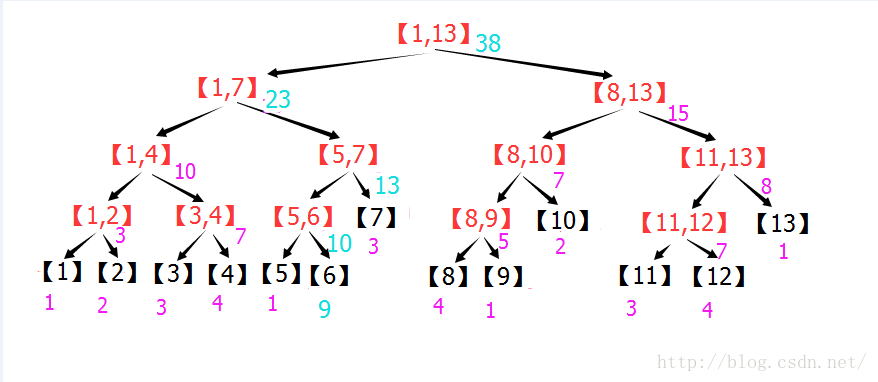# SegmentTree

SegmentTree（线段树）

## 引导

10000个正整数，编号从1到10000，用A,A,A表示。


• 不修改，直接统计：

方法一：对于统计L,R ，需要求下标从L到R的所有数的和，从L到R的所有下标记做[L..R],问题就是对A[L..R]进行求和。
这样求和，对于每个询问，需要将(R-L+1)个数相加。

方法二：更快的方法是求前缀和,令 S=0, S[k]=A[1..k] ，那么，A[L..R]的和就等于S[R]-S[L-1]，
这样，对于每个询问，就只需要做一次减法，大大提高效率。

• 先修改，后统计：

用方法二的话，假如A[L]+=C之后，S[L],S[L+1],...,S[R]都需要增加C,全部都要修改。

• 对比：

A[L]+=C 修改1个元素 （快） 修改R-L+1个元素

## 概念

• 时间复杂度 $O(logN)$
• 空间复杂度 $O(N)$

N 表示区间数量

## 结构

### 图示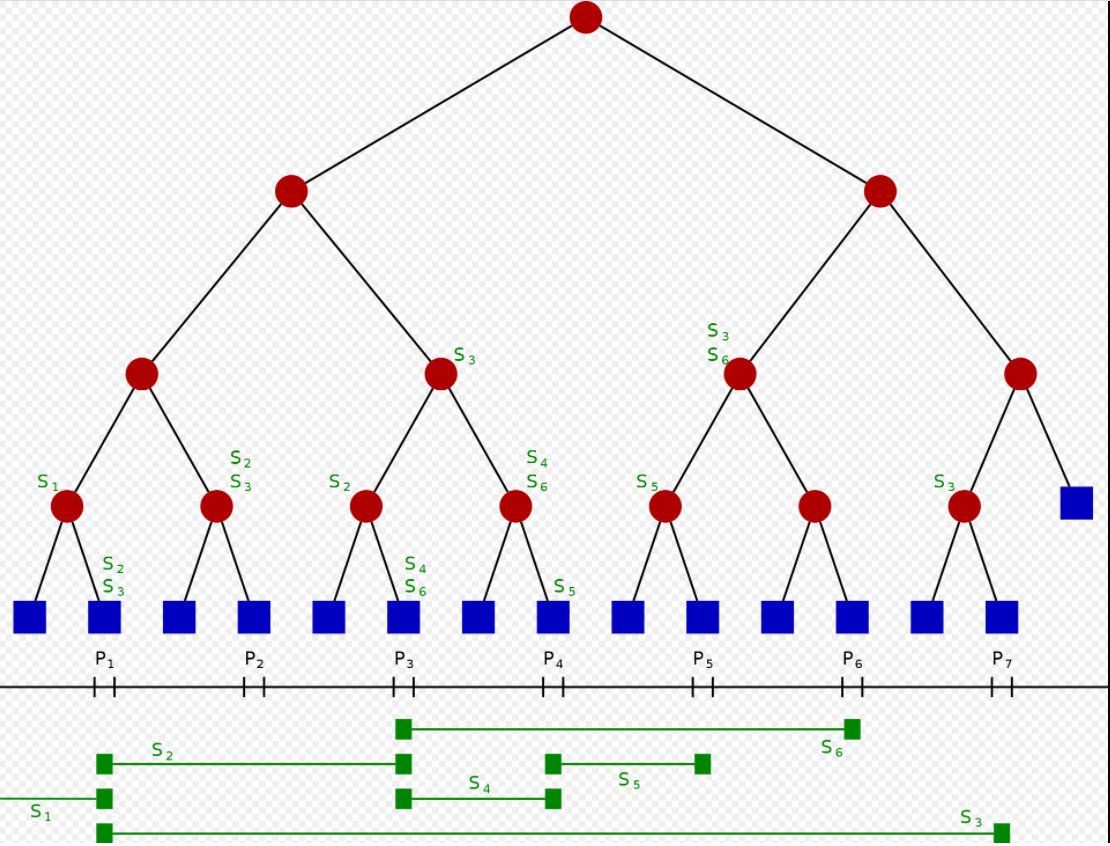单位区间：被端点切分的区间称为单位区间，每个端点所在位置也单独成为单位区间，比如：$(-\infty, x_1),[x_1,x_1],(x_1,x_2),[x_2,x_2],\dots,(x_{m-1},x_m),[x_m,x_m],(x_m,+\infty)$

其每一个叶节点，从左到右代表每个单位区间。



### 示例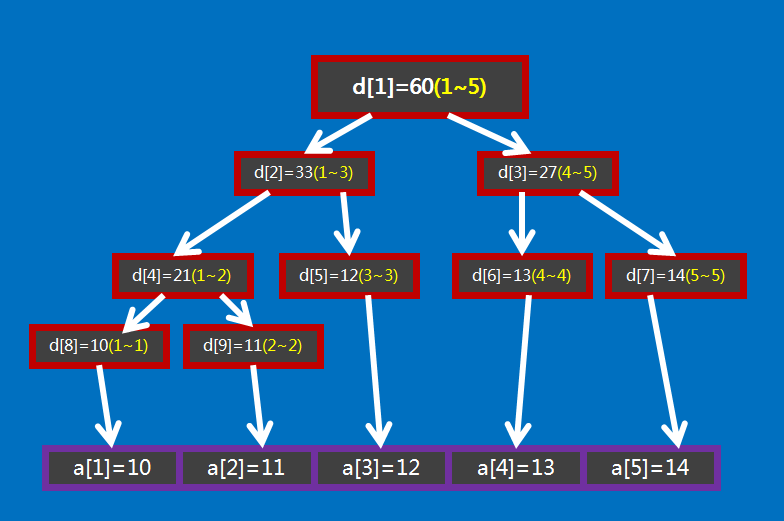$d_1$ 所保存的值是 $d_1 = 60 = a1+...+a5$

### 发现基本规律

1. $d_i$ 的左孩子是 $d_{2i}$ , 右孩子是 $d_{2i+1}$；

2. 如果用 $d_i$ 表示区间 $[s,t]$ 的值, 那么其左孩子表示区间 $[s, \frac{s+t}{2}]$, 右孩子表示区间$[\frac{s+t}{2}+1, t]$

3. 如果$d_i$表示的区间大小等于$1$, 那么其区间 $[s,t]$ 中肯定有 $s=t$, 且 $d_i = a_s = a_t$ , 即叶节点，这也就是线段树的递归边界。

## 操作

### 建树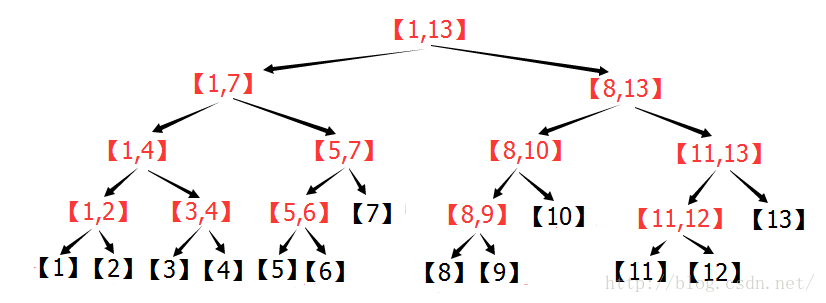void build(int s, int t, int p) {
// 对 [s,t] 区间建立线段树,当前根的编号为 p
if (s == t) {  // 规律3
d[p] = a[s];
return;
}
int m = (s + t) / 2;
build(s, m, p * 2), build(m + 1, t, p * 2 + 1);  // 规律2
// 递归对左右区间建树
d[p] = d[p * 2] + d[(p * 2) + 1];  // 规律1
}


### 展开子区间

#### 自下而上-利于理解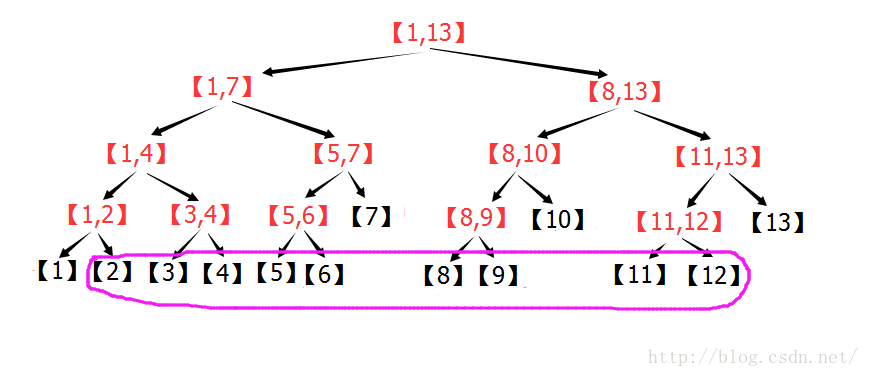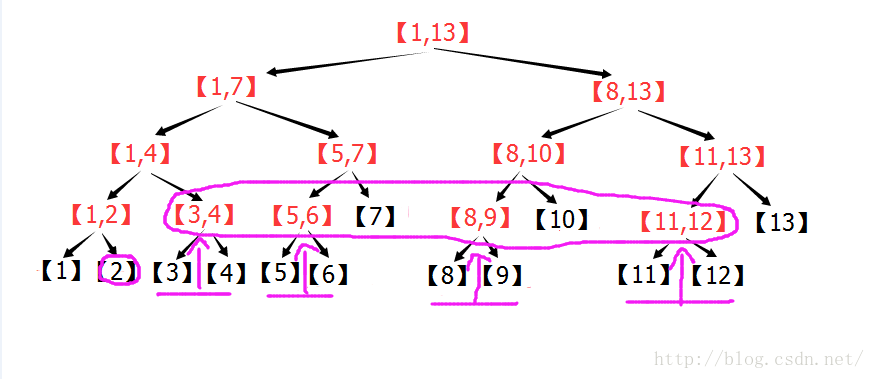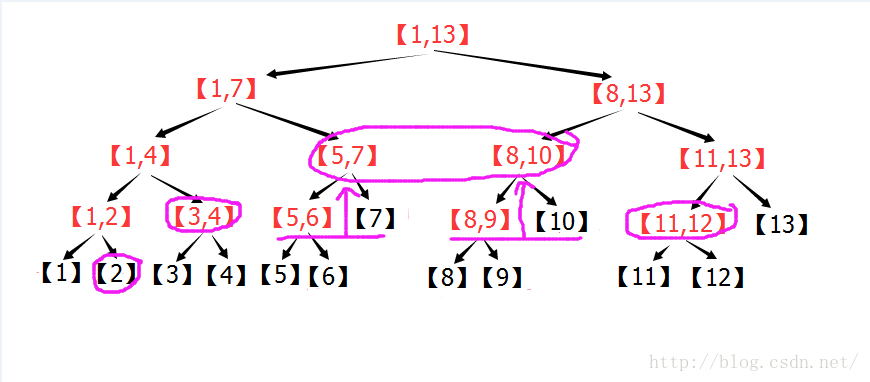#### 自上而下-利于计算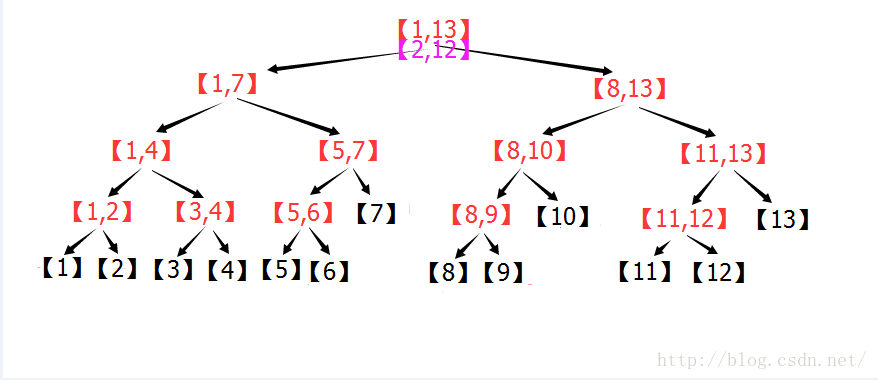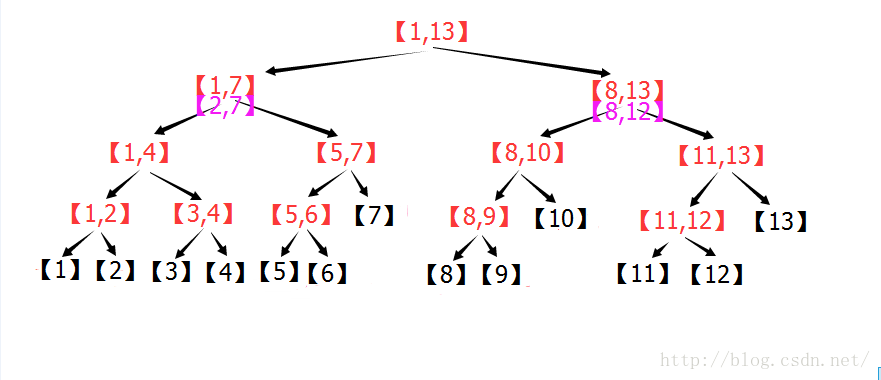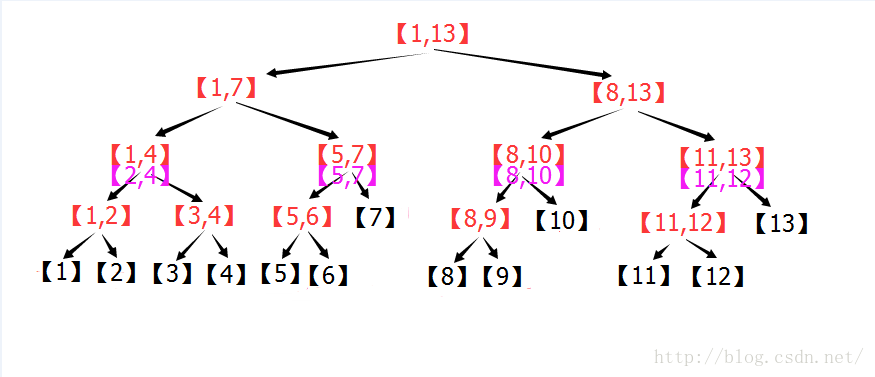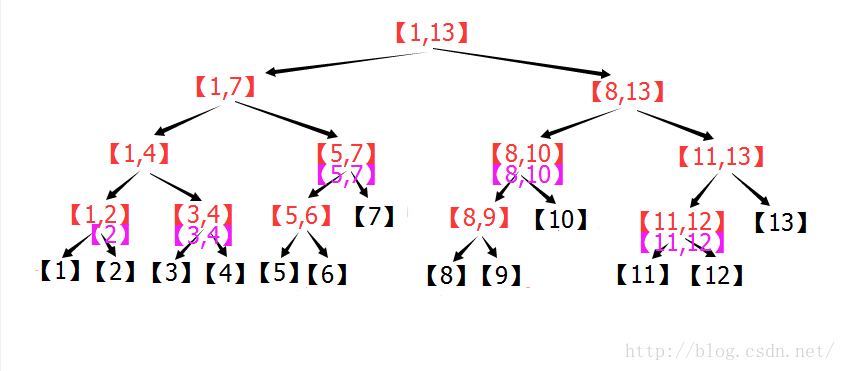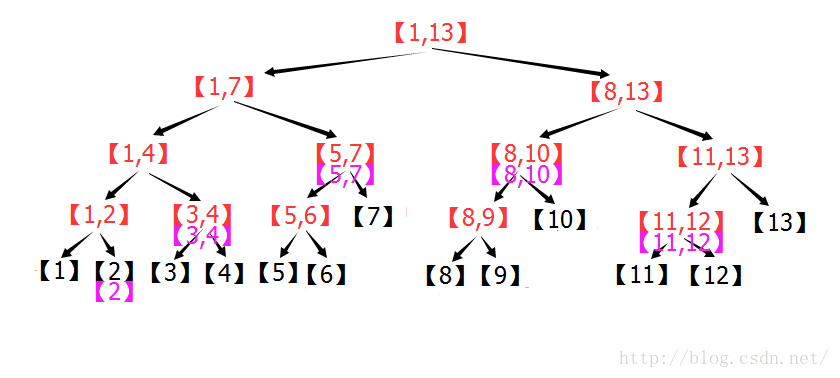### 区间查询/统计

1. 当前结点所代表的区间完全位于给定需要被查询的区间之外，则不应考虑当前结点
2. 当前结点所代表的区间完全位于给定需要被查询的区间之内，则可以直接查看当前结点的父结点
3. 当前结点所代表的区间部分位于需要被查询的区间之内，部分位于其外，则我们先考虑位于区间外的部分，后考虑区间内的（总有可能找到完全位于区间内的结点，因为叶子结点的区间长度为1，因此我们总能组合出合适的区间）

    int getsum(int l, int r, int s, int t, int p) {
// [l,r] 为查询区间,[s,t] 为当前节点包含的区间,p 为当前节点的编号
if (l <= s && t <= r)
return d[p];  // 当前区间为询问区间的子集时直接返回当前区间的和
int m = (s + t) / 2, sum = 0;
if (l <= m) sum += getsum(l, r, s, m, p * 2);
// 如果左儿子代表的区间 [l,m] 与询问区间有交集,则递归查询左儿子
if (r > m) sum += getsum(l, r, m + 1, t, p * 2 + 1);
// 如果右儿子代表的区间 [m+1,r] 与询问区间有交集,则递归查询右儿子
return sum;
}


### 单点修改